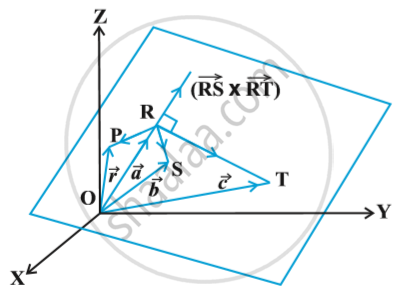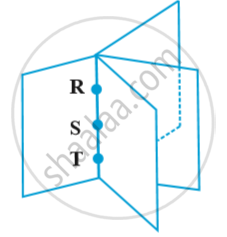# Plane - Equation of a Plane Passing Through Three Non Collinear Points

#### notes

Let R, S and T be three non collinear points on the plane with position vectors vec a , vec b and vec c respectively in following fig.The vectors vec (RS) and vec (RT) are in the given plane. Therefore , the vector vec (RS) xx vec (RT) is perpendicular to the plane containing points R,S and T. Let vec rbe the position vector of any point P in the plane.  Therefore, the equation of the plane passing through R and  perpendicular to the vector  vec (RS) xx vec (RT) is
(vec r - vec a) . (vec (RS) xx vec (RT)) = 0
or (vec r - vec a) . [(vec b - vec a) xx (vec c - vec a)] = 0  ...(1)
If the three points were on the same line, then there will be many planes that will contain them Fig.for example , These planes will resemble the pages of a book where the line containing the points R, S and T are members in the binding of the book.

Cartesian form:
Let (x_1,y_1,z_1) , (x_2 , y_2 , z_2) and (x_3 , y_3 , z_3)  be the coordinates of the points R, S and T respectively.  Let (x, y, z) be the coordinates of any point P on the plane with position vector vec r. Then
vec (RP) = (x - x_1) hat i + ( y - y_1) hat j + (z - z_1) hat k
vec (RS) = (x_2 - x_1) hat i + ( y_2 - y_1) hat j + (z_2 - z_1) hat k
vec (RT) = (x_3 - x_1) hat i + ( y_3 - y_1) hat j + (z_3 - z_1) hat k
Substituting these values in equation (1) of the vector form and expressing it in the form of a determinant, we have
|(x - x_1 , y - y_1 , z - z_1),(x_2 - x_1 , y_2 - y_1, z_2 -z_1) ,(x_3 - x_1, y_3 - y_1, z_3 - z_1) | = 0
which is the equation of the plane in Cartesian form passing through three non collinear points (x_1, y_1, z_1), (x_2, y_2, z_2) and (x_3, y_3, z_3).

If you would like to contribute notes or other learning material, please submit them using the button below.

#### Video Tutorials

We have provided more than 1 series of video tutorials for some topics to help you get a better understanding of the topic.

Series 1

Series 2

### Shaalaa.com

Equation of a Plane Passing Through Three Non-Collinear Points [00:03:44]
S
0%